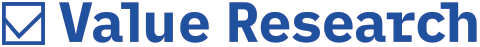# The Ben Graham Way

##### We tweak Benjamin Graham's simple formula for finding approximate valuations for growth stocks to make it work for Indian investors

Every investor faces the question whether a scrip is overpriced, underpriced or fairly priced. It's generally not possible for retail/lay investors to carry out long, intensive mathematical calculations using various valuation models to figure this out. So, what should he do? Benjamin Graham's simple formula for finding valuations of growth stocks comes to the rescue. Benjamin Graham mentions the formula in his famous books Security Analysis and The Intelligent Investor.
Following is the Benjamin Graham formula:

Intrinsic value = Earnings per share ×
[(8.5 + (2 × Expected annual growth rate, g)]

The earnings per share is the trailing twelve-month earnings. 8.5 is the P/E base for a no-growth company. Expected annual growth rate is the estimated growth rate over seven to ten years.
In 1974, in the revised edition of The Intelligent Investor, Graham revised the formula to

Intrinsic value = [EPS × (8.5 + 2g) × 4.4]/Y

In this formula, 4.4 is the then prevailing (1962) rate on high-grade corporate bonds listed on the New York Stock Exchange. Y is the current yield on AAA-rated corporate bonds.

Graham thought that as the investor had the choice between putting money in common stocks or bonds, it was appropriate to take into account the rate of interest paid on a high-grade bond - 4.4 per cent- in determining the intrinsic value of a stock.

Dividing by the current yield on the AAA-rated (Y) corporate bonds normalises the 4.4 per cent bond rate to today's environment. The reason for the inclusion of Y is that Graham wanted a minimum required rate of return for investing in stocks. As you test the formula yourself, you will notice that bond rates affect valuation. The lower the yield the higher the price. This fact goes back to bond basics. If the yield is low, the price is high. If the yield is high, the price is low. Graham designed the formula to replicate this line of thought.

Since all intrinsic value calculations and formulas are based upon the opportunity cost relative to the risk-free interest rate, the modified formula has incorporated the same.

Tweaking the formula as per Indian markets
The formula requires minor tweaking to make it work as per the Indian market and to match it with the current time and scenario.

Instead of using 8.5 as the no-growth P/E, we will use a P/E of 7 in our version of the tweaked formula. This is because even if a company has zero growth prospects but it is able to maintain cash flows and distribute dividends, its P/E is generally higher than 8.5.

Instead of using a single figure for growth rate, calculate the average of five-six years of growth experienced by the company. If a company has only three years of operating history, then take the average of the three years.

Next is the '2' multiplier, which is too aggressive. This is understandable if you take things into perspective. Graham never experienced companies with growth rates of 15-25 per cent, which is common today. Instead of '2', you can reduce the multiplier to 1.5 or 1. From all the calculations we have performed using the Graham formula, we have found that using 1 is completely satisfactory and still yields an optimistic value.

Instead of multiplying by 4.4, the interest rate prevailing on high-grade bonds, which is basically the substitute of the risk-free return, we use five-year fixed deposit rate of 8.5 per cent.

The modified Graham formula is:

Intrinsic value = [EPS × (7 + g) × 8.5]/Y

Let's look at SBI's intrinsic value using the information mentioned above.
SBI's TTM EPS = 22.57
Annual growth rate = 9.55 per cent
Current yield on the AAA-rated
corporate bonds = 8.3
SBI's intrinsic value = [(22.57) × (7 + 9.55) × 8.5]/
8.3 = ₹377.53

Margin of safety
The difference between the intrinsic value and the current market price is the margin of safety. The greater the margin of safety the safer the investment. Warren Buffet recommends at least a margin of safety of 25 per cent.

Word of caution
This simple, easy-to-do calculation is no substitute for intensive, long and complex valuation models used by analysts. This formula won't give you a 'true' value but a value close to the valuation model. Do not follow it blindly.

We have compiled for you the intrinsic valuation of select Sensex companies and their margin of safety.

# Overvalued sensex shares

 Company CMP (₹) Intrinsic Value (₹) Margin of Safety HDFC 1244.75 1243.8 -0.0008 NTPC 150.15 144.73 -0.0374 ITC 347.15 329.1 -0.0548 GAIL (India) 369.1 339.24 -0.088 Tata Steel 370.15 330.35 -0.1205 Oil & Natural Gas 315.8 275.25 -0.1473 Maruti Suzuki India 3539.8 2303.97 -0.5364 Hero MotoCorp 2352.55 1417.52 -0.6596 Larsen & Toubro 1679.6 985.53 -0.7043 Hindustan Unilever 889.55 460.7 -0.9309 Cipla 637.8 190.44 -2.3491

# Undervalued sensex shares

 Company CMP (₹) Intrinsic Value (₹) Margin of Safety Sesa Sterlite 205.05 856 0.7605 Tata Motors 515.3 1225.11 0.5794 ICICI Bank 308.1 594.87 0.4821 Axis Bank 527.55 967.01 0.4545 Sun Pharmaceutical Industries 947.8 1644.24 0.4236 Bajaj Auto 1996.85 3207.44 0.3774 Tata Consultancy Services 2496.9 3944.88 0.3671 State Bank Of India 275.65 377.53 0.2699 Reliance Industries 879.4 1178.07 0.2535 Wipro 650.25 783.43 0.17 HDFC Bank 1244.75 1480.93 0.1595 Infosys 1996.25 2364.11 0.1556 Mahindra & Mahindra 1170.45 1374.23 0.1483